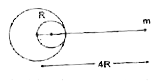Doubtnut App - Get 50 Lakh+ video solutions better than GoogleInstall Now

# A particle of mass m is placed at a distance of 4R from the centre of a huge uniform sphere of mass M and radius R. A spherical cavity of diameter R is made in the sphere as shown in the figure. If the gravitational interaction potential energy of the system of mass m and the remaining sphere after making the cavity is λGMm28R. Find the value of λText Solution
Verified by Experts

## PEnet=PEdue to entirc sphere−PEdue to removed portion PEnet=−GMm4R−[−GMm4R−(R/2)] but M.=M8(∴M∝R3)M.=(M/43πR3)43π(R2)3=M8 PEnet=−6GMm28R